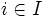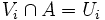# Compactness is weakly hereditary

This article gives the statement, and possibly proof, of a topological space property (i.e., compact space) satisfying a topological space metaproperty (i.e., weakly hereditary property of topological spaces)
View all topological space metaproperty satisfactions | View all topological space metaproperty dissatisfactions
Get more facts about compact space |Get facts that use property satisfaction of compact space | Get facts that use property satisfaction of compact space|Get more facts about weakly hereditary property of topological spaces

## Statement

### Property-theoretic statement

The property of topological spaces of being compact satisfies the metaproperty of being weakly hereditary: in other words, it is inherited by closed subsets.

### Verbal statement

Any closed subset of a compact space is compact (when given the subspace topology).

## Proof

### Proof in terms of open covers

Given:$X$ a compact space,$A$ a closed subset (given the subspace topology)

To prove: Consider an open cover of$A$ by open sets$U_i$ with$i \in I$, an indexing set. The$U_i$ have a finite subcover.

Proof:

Step no. Assertion/construction Given data used Facts used Previous steps used Explanation
1 By the definition of subspace topology, we can find open sets$V_i$ of$X$ such that$V_i \cap A = U_i$, thus the union of the$V_i$s contains$A$.$A$ is a subspace of$X$ -- --
2 The$V_i$s along with$X \setminus A$ form an open cover of$X$$A$ is closed in$X$ -- Step (1) [SHOW MORE]
3 The open cover from step (2) has a finite subcover. In other words, there is a finite subcollection of the$V_i$s, that, along with$X \setminus A$, covers$X$.$X$ is compact -- Step (2)
4 By throwing out$X \setminus A$, we get a finite collection of$V_i$s whose union contains$A$ -- -- Step (3)
5 The corresponding$U_i$ now form a finite subcover of the original cover of$A$. -- -- Steps (1), (4)

### Proof in terms of finite intersection property

Fill this in later